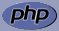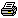www.PHPBuddy.comPHP Function Lookup:

 Categories
 PHP Quick Start PHP Installation PHP Articles PHP Scripts

 Top Rated Articles

 Site Related
 Submit Articles/Code Contact UsArticle Added on: April 12, 2002
Introducing FORMSOverview: Introduction to FORMS and how to pass information from one page to other

Okay till now we were working on single PHP pages and there was little interaction, take the example of the mulitplication table, woudn't it be great if the user could enter which multiplication table he wanted and then we could give him that result.

As PHP is a server side scripting language user interaction can only occur when we send some info to the server, in turn the server processes our request and sends the requested page, thus we need to post (Send) data to the server.

To illustrate I will show you an example: Let's build a multiplication table code where in the first page (ask_user.htm) we ask the user which table he wants and in the second page (table_results.php) we get that info and sends him the table.

 ask_user.htmView Sample Output

```<html> <body> <form method=post action=table_results.php> Enter the multiplication table number <input type=text name=table size=2> <input type=submit> </form> </body></html>```
What we have done in the above html page is that we have created a HTML FORM that is going to POST (send) data to the PHP file table_results.php this file will be called by the web-server and processed after processing it will be sent to the browser.

The PHP global array \$_POST['variable name'] which is a built in super global array in PHP contains all the data that is posted by a form, you just need to pass your variable name to retrive the data that you posted.

table_results.php

```<html><body> <?php \$cnt = 1; \$table = \$_POST['table']; while(\$cnt != 11) { echo "\$table x \$cnt = ". \$table * \$cnt; \$cnt++; } ?> </body></html>```

Analysisng the code: Enter any number in the above HTML form and press enter voila! you get the multiplication table for that, but how does the other programme table_results.php know which table to process.

Remember we have posted data to the server, see this line <input type=text name=table size=2> holds the value of the table, we have named the input field as table which now become our variable that stores the info.

We retrive the value of the table using \$_POST['variable_name'] in our case our variable that hold the value is table so we wrote the code \$table=\$_POST['table']; which retrives the value and stores it in the \$table variable.

Thus using forms we can pass data from one page to another. We can also pass information in URLS we do that using Query strings